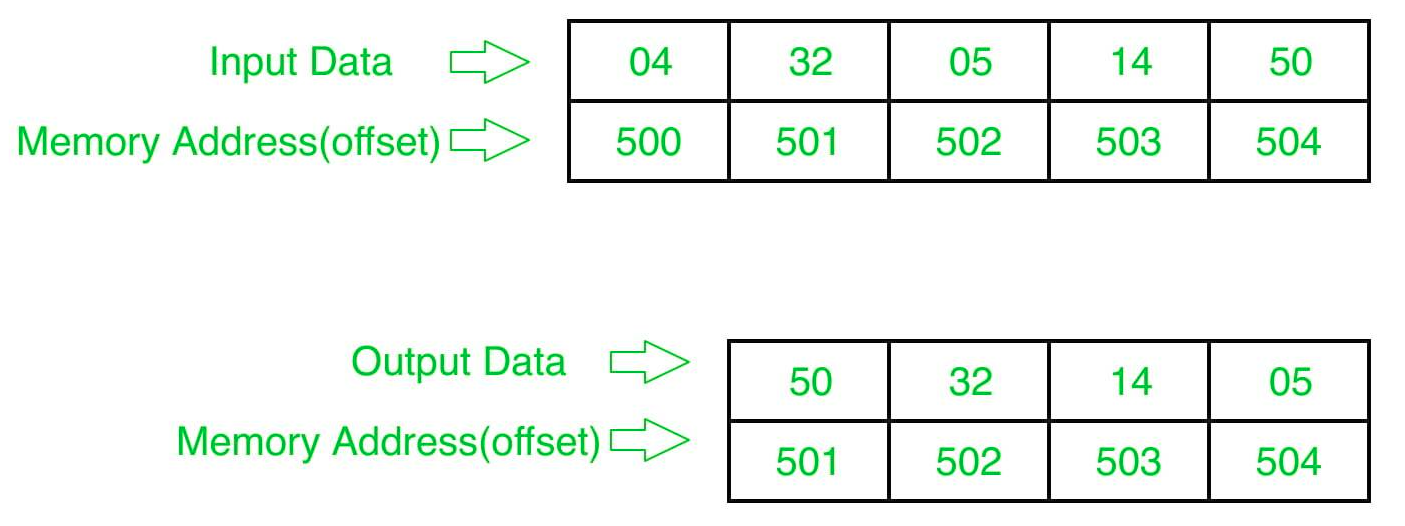# 8086 program to sort an integer array in descending order

Problem – Write a program in 8086 microprocessor to sort numbers in descending order in an array of n numbers, where size “n” is stored at memory address 2000 : 500 and the numbers are stored from memory address 2000 : 501.

Example –Example explanation:

```Pass-1:
32 05 14 50
32 05 14 50
32 14 05 50
32 14 50 05 (1 number got fix)

Pass-2:
32 14 50 05
32 14 50 05
32 50 14 05 (2 number got fix)

Pass-3:
32 50 14 05
50 32 14 05 (sorted)```

Algorithm –

1. Load data from offset 500 to register CL (for count).
2. Travel from starting memory location to last and compare two numbers if first number is smaller than second number then swap them.
3. First pass fix the position for last number.
4. Decrease the count by 1.
5. Again travel from starting memory location to (last-1, by help of count) and compare two numbers if first number is smaller than second number then swap them.
6. Second pass fix the position for last two numbers.
7. Repeate.

Program –

400 MOV SI, 500 SI<-500
403 MOV CL, [SI] CL<-[SI]
405 DEC CL CL<-CL-1
407 MOV SI, 500 SI<-500
40A MOV CH, [SI] CH<-[SI]
40C DEC CH CH<-CH-1
40E INC SI SI<-SI+1
40F MOV AL, [SI] AL<-[SI]
411 INC SI SI<-SI+1
412 CMP AL, [SI] AL-[SI]
416 XCHG AL, [SI] SWAP AL AND [SI]
418 DEC SI SI<-SI-1
419 XCHG AL, [SI] SWAP AL AND [SI]
41B INC SI SI<-SI+1
41C DEC CH CH<-CH-1
420 DEC CL CL<-CL-1
424 HLT END

Explanation –

1. MOV SI, 500: set the value of SI to 500.
2. MOV CL, [SI]: load data from offset SI to register CL.
3. DEC CL: decrease value of register CL BY 1.
4. MOV SI, 500: set the value of SI to 500.
5. MOV CH, [SI]: load data from offset SI to register CH.
6. DEC CH: decrease value of register CH BY 1.
7. INC SI: increase value of SI BY 1.
8. MOV AL, [SI]: load value from offset SI to register AL.
9. INC SI: increase value of SI BY 1.
10. CMP AL, [SI]: compares value of register AL and [SI] (AL-[SI]).
12. XCHG AL, [SI]: exchange the contents of register AL and SI.
13. DEC SI: decrease value of SI by 1.
14. XCHG AL, [SI]: exchange the contents of register AL and SI.
15. INC SI: increase value of SI by 1.
16. DEC CH: decrease value of register CH by 1.
18. DEC CL: decrease value of register CL by 1.